# 9. Theory of Graph Neural Networks

tobigsgnn1415·2021년 9월 6일
4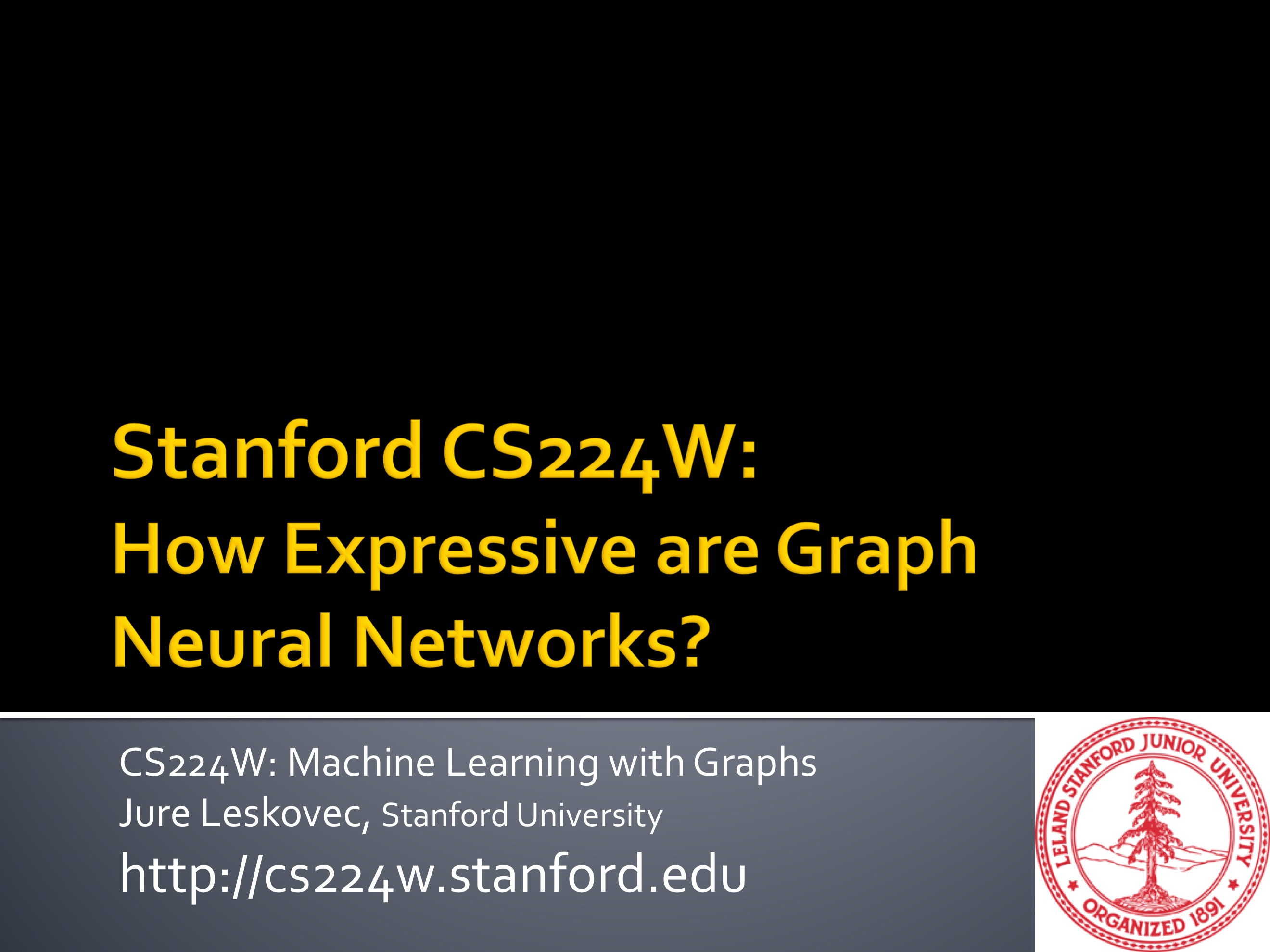작성자 : 장아연

# Aggregate Neighbors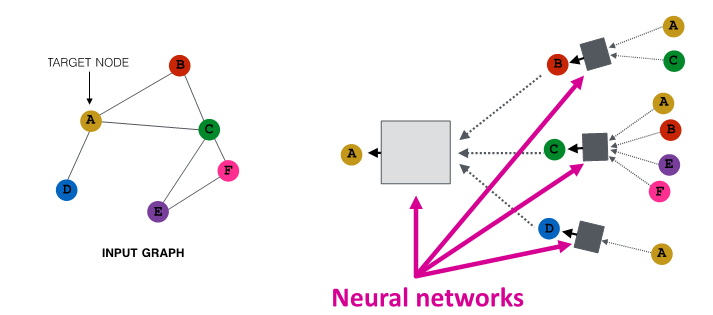• local network neighborhood를 바탕으로 node embedding 생성
• node는 neural network 이용해 neighbor로부터 aggregate information

# GNN Model

• 서로 다른 GNN model은 서로 다른 neural network를 사용
GCN (mean - pool)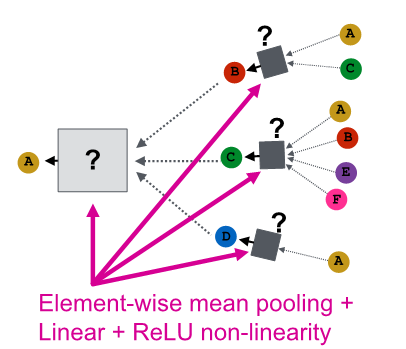GraphSAGE (max - pool)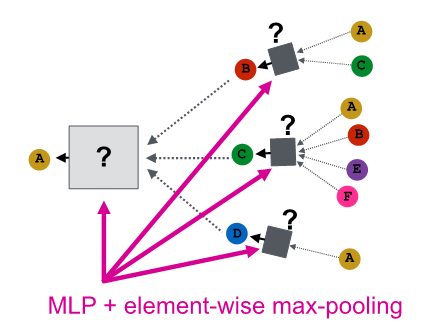# Local Neighborhood structure

Note : Node Color
use color to represent node feature
-> same color = same feature representation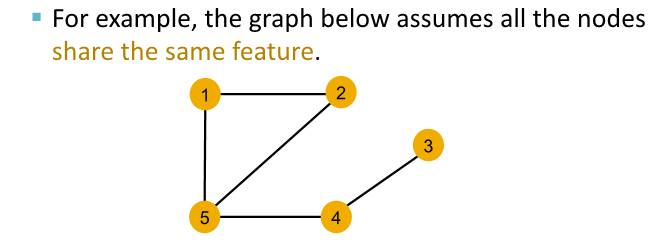Can GNN distinguish different graph structure?

• quantify local network neighborhood around each node

easy to distinguish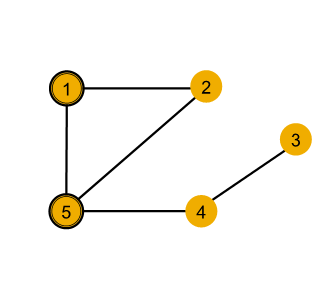nodesingle hop neighborhood
node 1degree of 2
node 5degree of 3

case 1 : hard to distinguish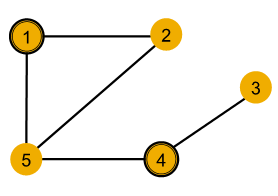nodesingle hop neighborhoodsecond hop neighborhood
node 1degree of 2degrees of 2 (node 2) and 3 (node 5)
node 4degree of 2degrees of 1 (node 3) and 3 (node 5)

case 2 : can not to distinguish

• symmetric within graph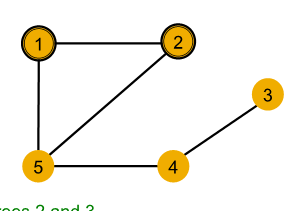nodesingle hop neighborhoodsecond hop neighborhood
node 1degree of 2degrees of 2 (node 2) and 3 (node 5)
node 2degree of 2degrees of 2 (node 1) and 3 (node 5)

go steep deeper to 2nd hop neighbor, still have same degree

# Computational Graph

• GNN aggregate neighbor node embedding
• define neighborhood
-> use computation graph
-> then GNN generate node embedding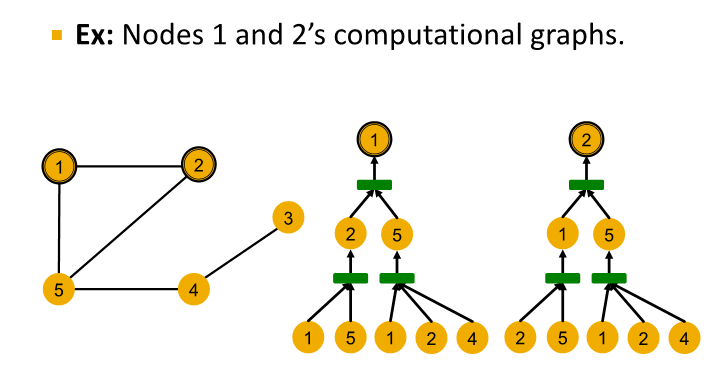• Node 1's commputational graph (2-layer GNN)
level 0 : (node 1, node 5) , (node 1, node 2, node 4)
level 1 :
node 5 aggregate information from (node 1, node 2, node 4)
node 2 aggregate information from (node 1, node 5)
level 2 :
node 1 aggregate information from node 2 and node 5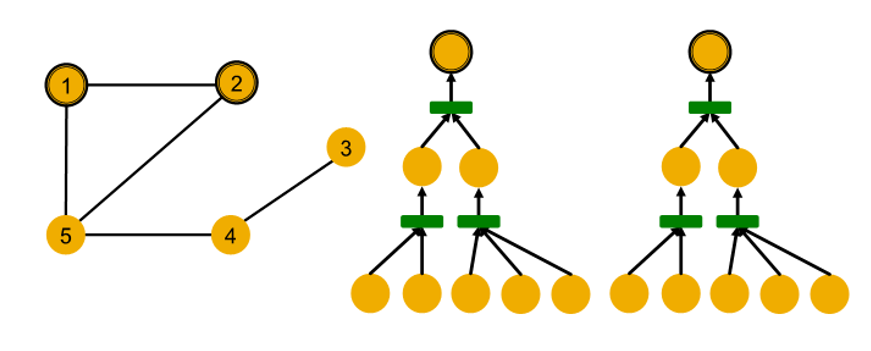• GNN은 message passing information without node ID, only use node feature

• computation graph of two node are same
-> embedded exactly into same point in embedding space

• GNN generate same embedding for node 1 and node 2
why ?
1. computational graph are same
2. node feature are same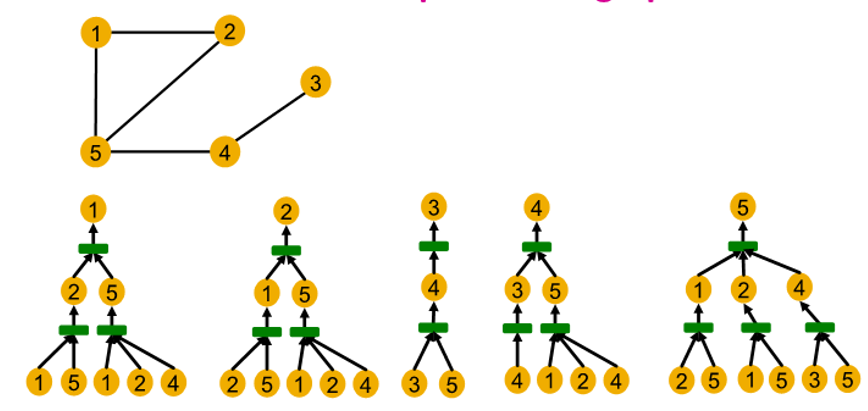• Computational graph are identical to rooted subtree structure for each node
• Rooted subtree structure
: recursively unfolding neighboring node from rooted nodes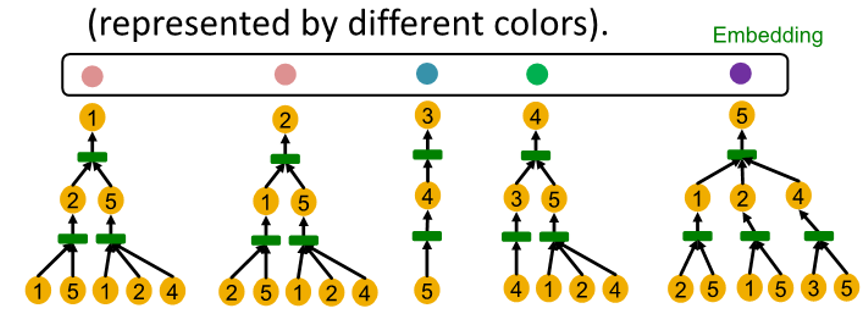• GNN은 rooted subtree structure 포착해 이에 따른 node embedding 매핑

# How expressive is a GNN?

Injective function

• $f$ : $X$ -> $Y$ : 서로 다른 X에 대해 서로 다른 Y 매핑
• $f$가 input $X$에 대한 모든 결과값을 가짐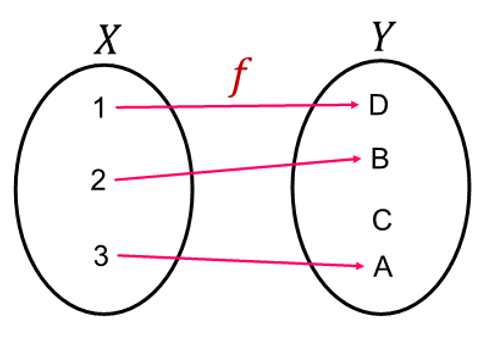GNN map subtree to node embedding injectively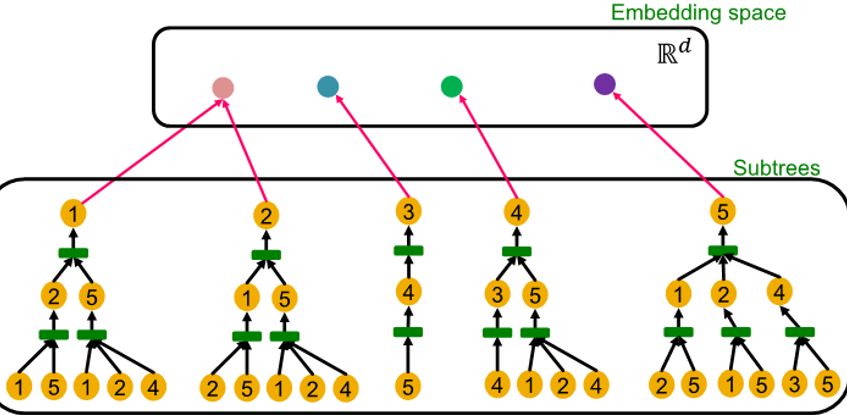Most Expressive GNN : use injective neighbor aggregation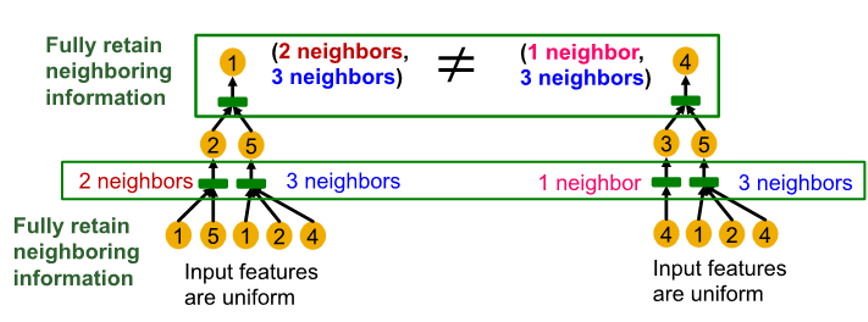• same depth subtree : recursively characterized from leaf to root node
• Injective neighbor aggregation :
서로 다른 neighbor -> 서로 다른 embedding
( GNN aggregation에서 neighborhood information을 완전히 얻는 경우, 생성된 node embedding은 서로 다른 rooted subtree 구별 가능 )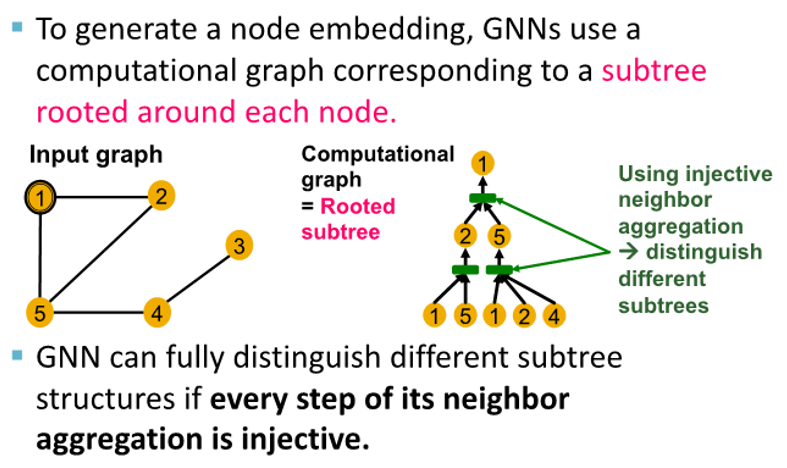# Neighbor Aggregation

• GNN 표현력은 사용하는 neighbor aggregation function에 따라 좌우됨

Neighbor Aggregation : a function over multi-set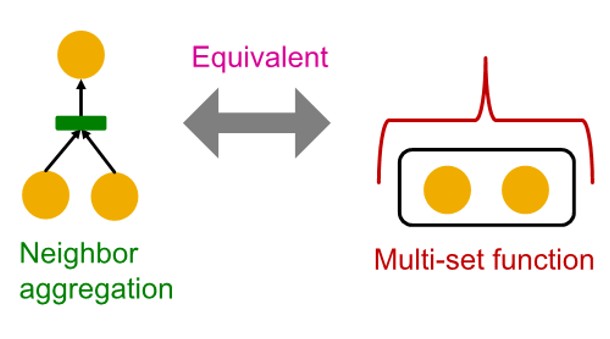neighbor aggregation
: node and aggregated from two neighbor for two children
Multi-set function
: set of children (two yellow) and need to aggregate information from that set

Aggregation function for GNN model

GCN (mean-pool)

• element wise mean -> linear function -> Relu activation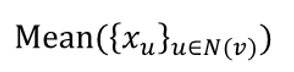• same color proportion의 multi-set 구별 불가능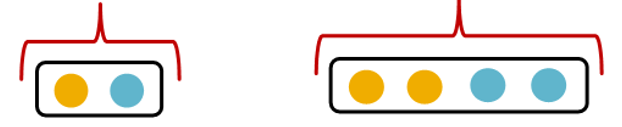• failure case example
represent node color by one-hot encoding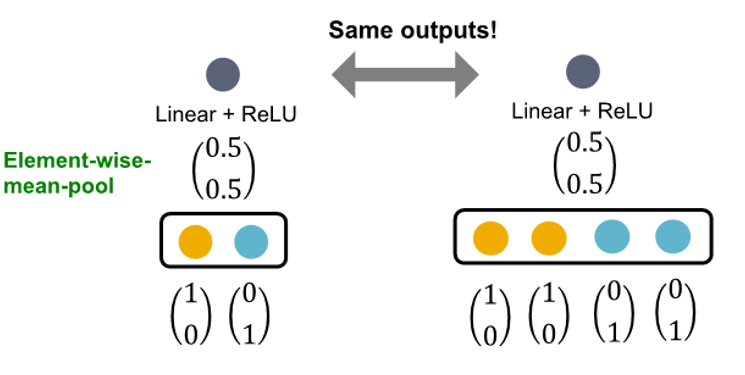GraphSAGE(max-pool)

• element wise max pooling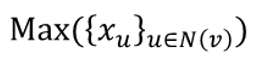• same distinct color set의 multi-set 구별 불가능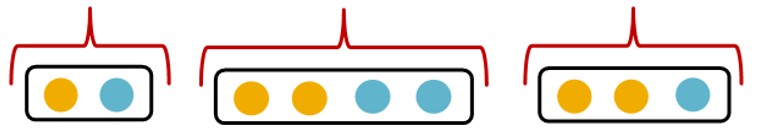• failure case examplenot injective : fail to distinguish some basic multi-set
not maximally powerfull GNN
need to design neural network that can model injective multiset function

# Injective Multi-Set Function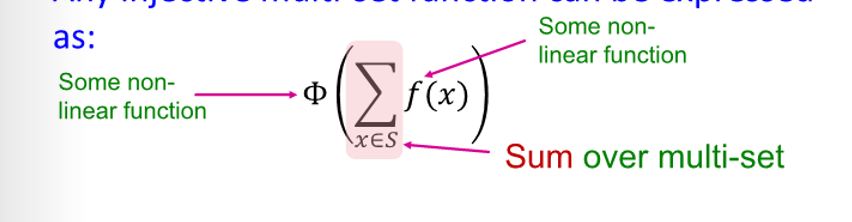• $f$ produce one-hot encoding of color
• summation of one-hot encoding retain all information about input multi-set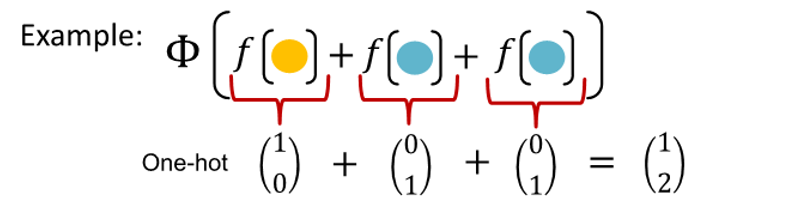# Universal Approximation Theorem

• use MLP(Multi-layer perceptron) to model $\Phi$ & $f$
• large hidden dimensionality 1-hidden layer MLP and non linearity
-> continous function을 arbitrary accuracy로 근사 가능
(hidden dimensionality는 100~500 정도가 적절)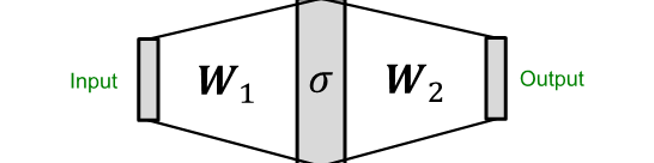# The Complete Graph Isomorphism Network Model• take multi-set with element $x$ -> apply multi-layer perceptron -> sum up -> apply another perceptron
• multi-layer perceptron은 임의의 함수에 근사하고 이로서 $f$$Phi$에도 근사됨
• GIN의 neighbor aggregation function은 injective함
GNN에서 가장 표현력이 좋음 & 실패 사례 없음

Full model of GIN with relation to WL Graph Kernel
color refinement algorithm in WL kernel

• initial color : $C^{(0)}(v)$ to each node
• interatively refine node color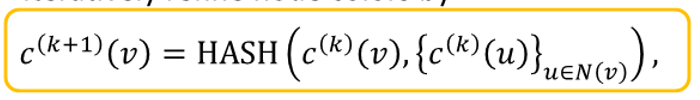to create color at k+1 for given node
-> take color of node $u$ that are its neighbor from previous iteration
-> then, take node $v$ from previous iteration
-> after, hash two colors
• after $K$ color refinement, $c^{(K)}(v)$$K$-hop neighborhood의 구조를 요약함

Example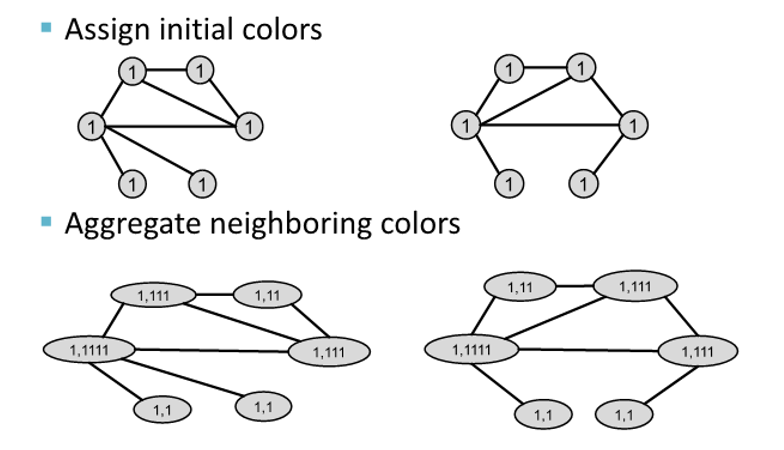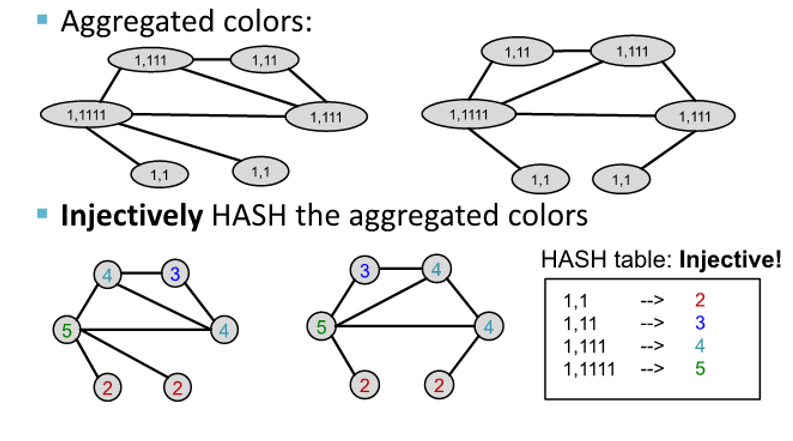• stable coloring에 도달할 때까지 process가 이어짐
• 두 그래프가 같은 color set을 가진 경우, isomorphic하다고 함

GIN use Neural Network to model injective Hash Function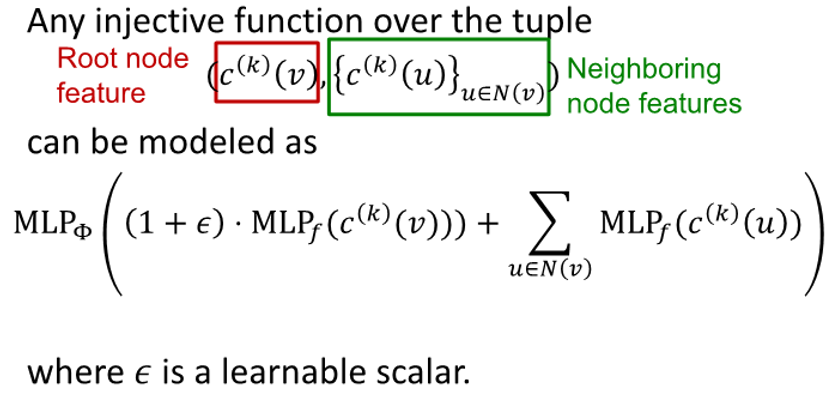• $(1 + \epsilon)$
• $MLP_{f}(c^{(k)}(v))$
: our own message transformed by $k$
• $\sum\limits_{u\in\mathbb{N(v)}} MLP_f(c^{(k)}(u))$
: take message from children and transform them using MLP, then sum up
• $MLP_{\Phi}($ $(1 + \epsilon)$ * $MLP_{f}(c^{(k)}(v))$ + $\sum\limits_{u\in\mathbb{N(v)}} MLP_f(c^{(k)}(u))$ $)$
: add two together and pass through another function $\Phi$

input feature가 one-hot vector로 표현 가능한 경우, direct summation은 injective하기 때문에 $\Phi$가 injective하도록 보장하면 됨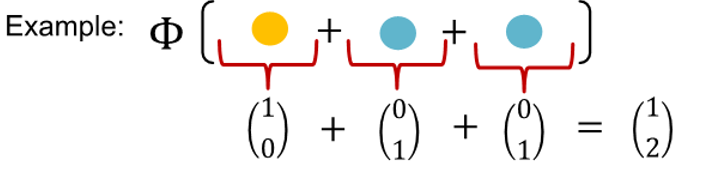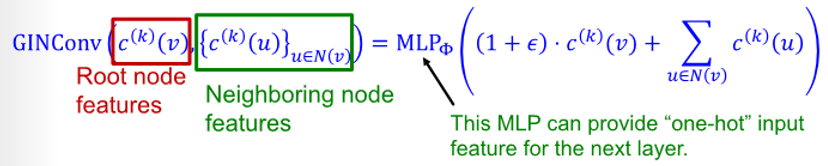GIN's node embedding update

• initial vector : $C^{(0)}(v)$ to each node
• interatively update node vector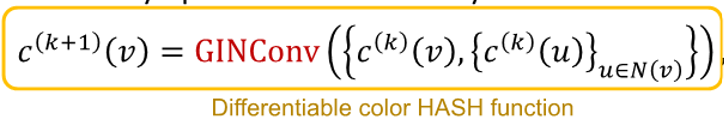• after $K$ step of GIN iteration, $c^{(K)}(v)$$K$-hop neighborhood의 구조를 요약함

# GIN and WL Kernel

GIN ~ differentiable neural network version of WL graph kernel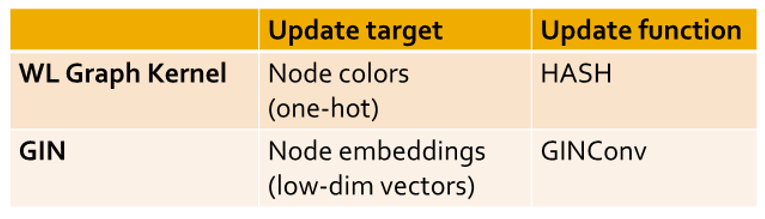• low dimensional of node embedding -> can capture fine-grained similarity of different node
• parameter of update function can be learned for the downstream task

GIN과 WL graph kernel의 연관성으로 인해 표현력은 동일
GIN은 실제 모든 graph를 구별 가능

# Power of Pooling

Failure case of mean and max pooling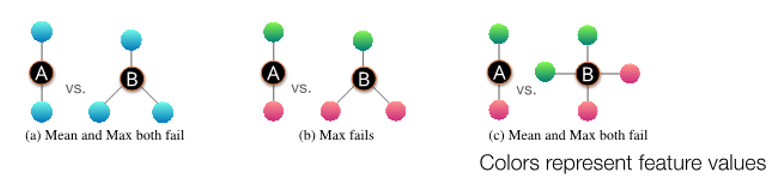(a) : type of neighborhood structure with all same feature
(b) : number of distinct color are same
(c) : proportion of distinct color is same

Ranking by discriminative power#### 3개의 댓글

14기 김상현

• GNN은 rooted subtree의 구조를 포착해 이에 따른 node embedding 수행
• Node feature가 같은 경우 computational graph가 같은 경우 injective node embedding 불가
• 표현력이 풍부한 GNN은 injective neighbor aggregation을 사용. 기존의 GCN, GraphSAGE는 not injective neighbor aggregation -> 표현력이 부족
• Injective aggregation을 위해 MLP을 이용 -> GIN(graph isomorphism network
• GIN은 WL graph kernel과 동일한 표현력을 갖으면서 미분 가능한 신경망
- GIN: GINConv를 사용해 node embeddings(low-dim vectors)
- WL graph kernel: Hash function 사용해 node colors(one-hot)

GNN의 표현력과 GIN에 대해 이해할 수 있었습니다.
유익한 강의 감사합니다 :)

답글 달기

15기 김재희

• GNN의 aggregation function은 이웃 노드에 대한 subtree 구조를 입력으로 하여 임베딩 벡터를 아웃풋으로 내보내는 함수
• message passing의 관점에서 표현력이 좋은 gnn이 되기 위해서는 aggregation function이 단사함수여야 함.
• 기존에 배웟던 mean, max pooling 등은 단사함수가 아니기 때문에 좋은 aggregation function이 아님
• MLP가 충분히 큰 hidden node를 가지면 어떤 연속함수든 근사가 가능하다는 성질을 이용하여 MLP로 aggregation function을 구성하면 단사함수가 됨.
• 이를 이용한 것이 GIN으로 수식적으로 WL kernel의 근사함수.
• 그러므로 GIN의 표현력의 상한은 wl kernel이 됨
답글 달기